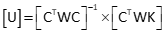## 3. The Weight Matrix

Equation E-4 is the general matrix algorithm for observation equations with weight matrix, [W], included; Equation E-5 is the matrix solution for the unknowns.Equation E-4Equation E-5

Because each observation has a weight, [W] is a diagonal m x m matrix, Figure E-5.Figure E-5 Weight Matrix

The iwi element is the weight for the ith observation. Off-diagonal elements are zero.

Since the weight matrix appears on both sides of Equation E-4, it cancels when computing the residuals, Equation E-6.Equation E-6

Weights are used in the standard deviation of the unit weight computation, Equation E-7.Equation E-7